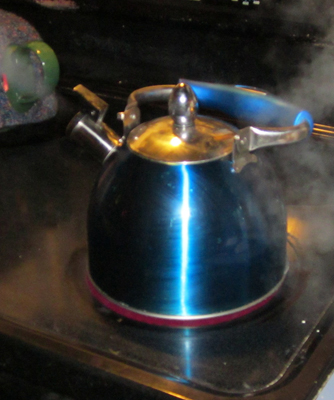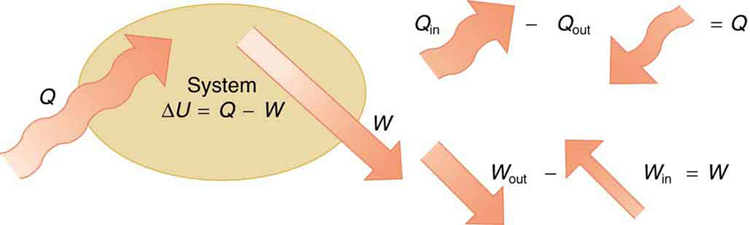# 15.1 The first law of thermodynamics

 Page 1 / 9
• Define the first law of thermodynamics.
• Describe how conservation of energy relates to the first law of thermodynamics.
• Identify instances of the first law of thermodynamics working in everyday situations, including biological metabolism.
• Calculate changes in the internal energy of a system, after accounting for heat transfer and work done.This boiling tea kettle represents energy in motion. The water in the kettle is turning to water vapor because heat is being transferred from the stove to the kettle. As the entire system gets hotter, work is done—from the evaporation of the water to the whistling of the kettle. (credit: Gina Hamilton)

If we are interested in how heat transfer is converted into doing work, then the conservation of energy principle is important. The first law of thermodynamics applies the conservation of energy principle to systems where heat transfer and doing work are the methods of transferring energy into and out of the system. The first law of thermodynamics    states that the change in internal energy of a system equals the net heat transfer into the system minus the net work done by the system. In equation form, the first law of thermodynamics is

$\Delta U=Q-W\text{.}$

Here $\Delta U$ is the change in internal energy $U$ of the system. $Q$ is the net heat transferred into the system —that is, $Q$ is the sum of all heat transfer into and out of the system. $W$ is the net work done by the system —that is, $W$ is the sum of all work done on or by the system. We use the following sign conventions: if $Q$ is positive, then there is a net heat transfer into the system; if $W$ is positive, then there is net work done by the system. So positive $Q$ adds energy to the system and positive $W$ takes energy from the system. Thus $\Delta U=Q-W$ . Note also that if more heat transfer into the system occurs than work done, the difference is stored as internal energy. Heat engines are a good example of this—heat transfer into them takes place so that they can do work. (See [link] .) We will now examine $Q$ , $W$ , and $\Delta U$ further.The first law of thermodynamics is the conservation-of-energy principle stated for a system where heat and work are the methods of transferring energy for a system in thermal equilibrium. Q size 12{Q} {} represents the net heat transfer—it is the sum of all heat transfers into and out of the system. Q size 12{Q} {} is positive for net heat transfer into the system. W size 12{W} {} is the total work done on and by the system. W size 12{W} {} is positive when more work is done by the system than on it. The change in the internal energy of the system, Δ U size 12{ΔU} {} , is related to heat and work by the first law of thermodynamics, Δ U = Q − W size 12{ΔU=Q - W} {} .

## Making connections: law of thermodynamics and law of conservation of energy

The first law of thermodynamics is actually the law of conservation of energy stated in a form most useful in thermodynamics. The first law gives the relationship between heat transfer, work done, and the change in internal energy of a system.

## Heat Q And work W

Heat transfer ( $Q$ ) and doing work ( $W$ ) are the two everyday means of bringing energy into or taking energy out of a system. The processes are quite different. Heat transfer, a less organized process, is driven by temperature differences. Work, a quite organized process, involves a macroscopic force exerted through a distance. Nevertheless, heat and work can produce identical results. For example, both can cause a temperature increase. Heat transfer into a system, such as when the Sun warms the air in a bicycle tire, can increase its temperature, and so can work done on the system, as when the bicyclist pumps air into the tire. Once the temperature increase has occurred, it is impossible to tell whether it was caused by heat transfer or by doing work. This uncertainty is an important point. Heat transfer and work are both energy in transit—neither is stored as such in a system. However, both can change the internal energy $U$ of a system. Internal energy is a form of energy completely different from either heat or work.

#### Questions & Answers

a15kg powerexerted by the foresafter 3second
Firdos Reply
what is displacement
Xolani Reply
movement in a direction
Jason
Explain why magnetic damping might not be effective on an object made of several thin conducting layers separated by insulation? can someone please explain this i need it for my final exam
anas Reply
Hi
saeid
hi
Yimam
What is thê principle behind movement of thê taps control
Oluwakayode Reply
what is atomic mass
thomas Reply
this is the mass of an atom of an element in ratio with the mass of carbon-atom
Chukwuka
show me how to get the accuracies of the values of the resistors for the two circuits i.e for series and parallel sides
Jesuovie Reply
Explain why it is difficult to have an ideal machine in real life situations.
Isaac Reply
tell me
Promise
what's the s . i unit for couple?
Promise
its s.i unit is Nm
Covenant
Force×perpendicular distance N×m=Nm
Oluwakayode
İt iş diffucult to have idêal machine because of FRİCTİON definitely reduce thê efficiency
Oluwakayode
if the classica theory of specific heat is valid,what would be the thermal energy of one kmol of copper at the debye temperature (for copper is 340k)
Zaharadeen Reply
can i get all formulas of physics
BPH Reply
yes
haider
what affects fluid
Doreen Reply
pressure
Oluwakayode
Dimension for force MLT-2
Promise Reply
what is the dimensions of Force?
Osueke Reply
how do you calculate the 5% uncertainty of 4cm?
melia Reply
4cm/100×5= 0.2cm
haider
how do you calculate the 5% absolute uncertainty of a 200g mass?
melia Reply
= 200g±(5%)10g
haider
use the 10g as the uncertainty?
melia
which topic u discussing about?
haider
topic of question?
haider
the relationship between the applied force and the deflection
melia
sorry wrong question i meant the 5% uncertainty of 4cm?
melia
its 0.2 cm or 2mm
haider
thank you
melia
Hello group...
Chioma
hi
haider
well hello there
sean
hi
Noks
hii
Chibueze
10g
Olokuntoye
0.2m
Olokuntoye
hi guys
thomas
the meaning of phrase in physics
Chovwe Reply
is the meaning of phrase in physics
Chovwe

### Read also:

#### Get Jobilize Job Search Mobile App in your pocket Now!

Source:  OpenStax, College physics. OpenStax CNX. Jul 27, 2015 Download for free at http://legacy.cnx.org/content/col11406/1.9
Google Play and the Google Play logo are trademarks of Google Inc.

Notification Switch

Would you like to follow the 'College physics' conversation and receive update notifications?ByBy Kimberly NicholsBy Frank LevyBy OpenStaxBy Brooke DelaneyBy JavaChamp TeamBy Sandhills MLTBy David MartinBy Keyaira BraxtonBy OpenStaxBy Mucho Mizinduko